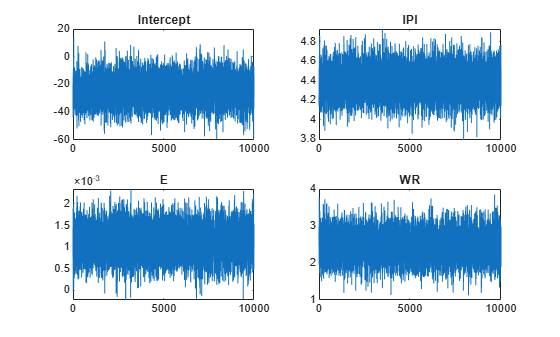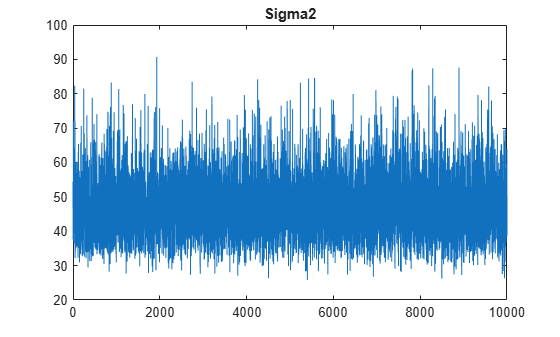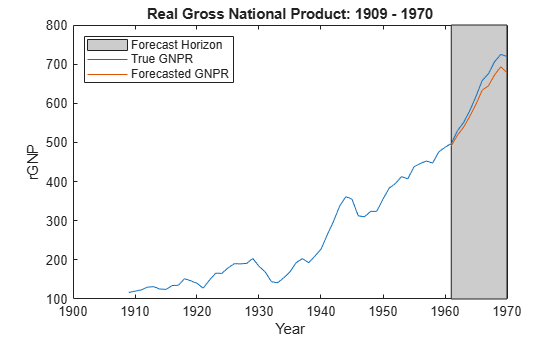# semiconjugateblm

Bayesian linear regression model with semiconjugate prior for data likelihood

## Description

The Bayesian linear regression model object `semiconjugateblm` specifies that the conditional prior distribution of β|σ2 is multivariate Gaussian with mean μ and variance V, and the prior distribution of σ2 is inverse gamma with shape A and scale B. Specifically, the Bayesian linear regression model is the independent, normal-inverse-gamma semiconjugate model.

The data likelihood is $\prod _{t=1}^{T}\varphi \left({y}_{t};{x}_{t}\beta ,{\sigma }^{2}\right),$ where ϕ(yt;xtβ,σ2) is the Gaussian probability density evaluated at yt with mean xtβ and variance σ2. The specified priors are semiconjugate for the likelihood, that is, the resulting conditional, but not marginal, posterior distributions are analytically tractable. For details on the posterior distribution, see Analytically Tractable Posteriors.

In general, when you create a Bayesian linear regression model object, it specifies the joint prior distribution and characteristics of the linear regression model only. That is, the model object is a template intended for further use. Specifically, to incorporate data into the model for posterior distribution analysis, pass the model object and data to the appropriate object function.

## Creation

### Syntax

``PriorMdl = semiconjugateblm(NumPredictors)``
``PriorMdl = semiconjugateblm(NumPredictors,Name,Value)``

### Description

example

````PriorMdl = semiconjugateblm(NumPredictors)` creates a Bayesian linear regression model object (`PriorMdl`) composed of `NumPredictors` predictors and an intercept. The joint prior distribution of (β, σ2) is the independent normal-inverse-gamma semiconjugate model. `PriorMdl` is a template defining the prior distributions and dimensionality of β.```

example

````PriorMdl = semiconjugateblm(NumPredictors,Name,Value)` uses additional options specified by one or more `Name,Value` pair arguments. `Name` is a property name, except `NumPredictors`, and `Value` is the corresponding value. `Name` must appear inside single quotes (`''`). You can specify several `Name,Value` pair arguments in any order as `Name1,Value1,...,NameN,ValueN`.```

## Properties

expand all

You can set property values when you create the model object using name-value pair argument syntax, or after model creation using dot notation. For example, to set a more diffuse prior covariance matrix for `PriorMdl`, a Bayesian linear regression model containing three model coefficients, enter

`PriorMdl.V = 100*eye(3);`

Number of predictor variables in the Bayesian multiple linear regression model, specified as a nonnegative integer.

`NumPredictors` must be the same as the number of columns in your predictor data, which you specify during model estimation or simulation.

When specifying `NumPredictors`, exclude any intercept term for the value.

After creating a model, if you change the of value `NumPredictors` using dot notation, then these parameters revert to the default values:

• Variable names (`VarNames`)

• Prior mean of β (`Mu`)

• Prior covariance matrix of β (`V`)

Data Types: `double`

Flag for including a regression model intercept, specified as a value in this table.

ValueDescription
`false`Exclude an intercept from the regression model. Therefore, β is a `p`-dimensional vector, where `p` is the value of `NumPredictors`.
`true`Include an intercept in the regression model. Therefore, β is a (`p` + 1)-dimensional vector. This specification causes a T-by-1 vector of ones to be prepended to the predictor data during estimation and simulation.

If you include a column of ones in the predictor data for an intercept term, then set `Intercept` to `false`.

Example: `'Intercept',false`

Data Types: `logical`

Predictor variable names for displays, specified as a string vector or cell vector of character vectors. `VarNames` must contain `NumPredictors` elements. `VarNames(j)` is the name of the variable in column `j` of the predictor data set, which you specify during estimation, simulation, or forecasting.

The default is `{'Beta(1)','Beta(2),...,Beta(p)}`, where `p` is the value of `NumPredictors`.

Example: `'VarNames',["UnemploymentRate"; "CPI"]`

Data Types: `string` | `cell` | `char`

Mean parameter of the Gaussian prior on β, specified as a numeric scalar or vector.

If `Mu` is a vector, then it must have `NumPredictors` or `NumPredictors + 1` elements.

• For `NumPredictors` elements, `semiconjugateblm` sets the prior mean of the `NumPredictors` predictors only. Predictors correspond to the columns in the predictor data (specified during estimation, simulation, or forecasting). `semiconjugateblm` ignores the intercept in the model, that is, `semiconjugateblm` specifies the default prior mean to any intercept.

• For `NumPredictors + 1` elements, the first element corresponds to the prior mean of the intercept, and all other elements correspond to the predictors.

Example: `'Mu',[1; 0.08; 2]`

Data Types: `double`

Conditional covariance matrix of Gaussian prior on β, specified as a `c`-by-`c` symmetric, positive definite matrix. `c` can be `NumPredictors` or ```NumPredictors + 1```.

• If `c` is `NumPredictors`, then `semiconjugateblm` sets the prior covariance matrix to

`$\left[\begin{array}{cccc}1e5& 0& \cdots & 0\\ 0& & & \\ ⋮& & V& \\ 0& & & \end{array}\right].$`

`semiconjugateblm` attributes the default prior covariances to the intercept, and attributes `V` to the coefficients of the predictor variables in the data. Rows and columns of `V` correspond to columns (variables) in the predictor data.

• If `c` is `NumPredictors + 1`, then `semiconjugateblm` sets the entire prior covariance to `V`. The first row and column correspond to the intercept. All other rows and columns correspond to the columns in the predictor data.

The default value is a flat prior. For an adaptive prior, specify ```diag(Inf(Intercept + NumPredictors,1))```. Adaptive priors indicate zero precision in order for the prior distribution to have as little influence as possible on the posterior distribution.

Example: `'V',diag(Inf(3,1))`

Data Types: `double`

Shape hyperparameter of the inverse gamma prior on σ2, specified as a numeric scalar.

`A` must be at least ```–(Intercept + NumPredictors)/2```.

With `B` held fixed, the inverse gamma distribution becomes taller and more concentrated as `A` increases. This characteristic weighs the prior model of σ2 more heavily than the likelihood during posterior estimation.

For the functional form of the inverse gamma distribution, see Analytically Tractable Posteriors.

Example: `'A',0.1`

Data Types: `double`

Scale parameter of inverse gamma prior on σ2, specified as a positive scalar or `Inf`.

With `A` held fixed, the inverse gamma distribution becomes taller and more concentrated as `B` increases. This characteristic weighs the prior model of σ2 more heavily than the likelihood during posterior estimation.

Example: `'B',5`

Data Types: `double`

## Object Functions

 `estimate` Estimate posterior distribution of Bayesian linear regression model parameters `simulate` Simulate regression coefficients and disturbance variance of Bayesian linear regression model `forecast` Forecast responses of Bayesian linear regression model `plot` Visualize prior and posterior densities of Bayesian linear regression model parameters `summarize` Distribution summary statistics of standard Bayesian linear regression model

## Examples

collapse all

Consider the multiple linear regression model that predicts U.S. real gross national product (`GNPR`) using a linear combination of industrial production index (`IPI`), total employment (`E`), and real wages (`WR`).

`${\text{GNPR}}_{t}={\beta }_{0}+{\beta }_{1}{\text{IPI}}_{t}+{\beta }_{2}{\text{E}}_{t}+{\beta }_{3}{\text{WR}}_{t}+{\epsilon }_{t}.$`

For all $t$ time points, ${\epsilon }_{t}$ is a series of independent Gaussian disturbances with a mean of 0 and variance ${\sigma }^{2}$.

Assume that the prior distributions are:

• $\beta |{\sigma }^{2}\sim {N}_{4}\left(M,V\right)$. $M$ is a 4-by-1 vector of means, and $V$ is a scaled 4-by-4 positive definite covariance matrix.

• ${\sigma }^{2}\sim IG\left(A,B\right)$. $A$ and $B$ are the shape and scale, respectively, of an inverse gamma distribution.

These assumptions and the data likelihood imply a normal-inverse-gamma semiconjugate model. That is, the conditional posteriors are conjugate to the prior with respect to the data likelihood, but the marginal posterior is analytically intractable.

Create a normal-inverse-gamma semiconjugate prior model for the linear regression parameters. Specify the number of predictors `p`.

```p = 3; Mdl = bayeslm(p,'ModelType','semiconjugate')```
```Mdl = semiconjugateblm with properties: NumPredictors: 3 Intercept: 1 VarNames: {4x1 cell} Mu: [4x1 double] V: [4x4 double] A: 3 B: 1 | Mean Std CI95 Positive Distribution ------------------------------------------------------------------------------- Intercept | 0 100 [-195.996, 195.996] 0.500 N (0.00, 100.00^2) Beta(1) | 0 100 [-195.996, 195.996] 0.500 N (0.00, 100.00^2) Beta(2) | 0 100 [-195.996, 195.996] 0.500 N (0.00, 100.00^2) Beta(3) | 0 100 [-195.996, 195.996] 0.500 N (0.00, 100.00^2) Sigma2 | 0.5000 0.5000 [ 0.138, 1.616] 1.000 IG(3.00, 1) ```

`Mdl` is a `semiconjugateblm` Bayesian linear regression model object representing the prior distribution of the regression coefficients and disturbance variance. At the command window, `bayeslm` displays a summary of the prior distributions.

You can set writable property values of created models using dot notation. Set the regression coefficient names to the corresponding variable names.

`Mdl.VarNames = ["IPI" "E" "WR"]`
```Mdl = semiconjugateblm with properties: NumPredictors: 3 Intercept: 1 VarNames: {4x1 cell} Mu: [4x1 double] V: [4x4 double] A: 3 B: 1 | Mean Std CI95 Positive Distribution ------------------------------------------------------------------------------- Intercept | 0 100 [-195.996, 195.996] 0.500 N (0.00, 100.00^2) IPI | 0 100 [-195.996, 195.996] 0.500 N (0.00, 100.00^2) E | 0 100 [-195.996, 195.996] 0.500 N (0.00, 100.00^2) WR | 0 100 [-195.996, 195.996] 0.500 N (0.00, 100.00^2) Sigma2 | 0.5000 0.5000 [ 0.138, 1.616] 1.000 IG(3.00, 1) ```

Consider the linear regression model in Create Normal-Inverse-Gamma Semiconjugate Prior Model.

Create a normal-inverse-gamma semiconjugate prior model for the linear regression parameters. Specify the number of predictors `p` and the names of the regression coefficients.

```p = 3; PriorMdl = bayeslm(p,'ModelType','semiconjugate','VarNames',["IPI" "E" "WR"]);```

Load the Nelson-Plosser data set. Create variables for the response and predictor series.

```load Data_NelsonPlosser X = DataTable{:,PriorMdl.VarNames(2:end)}; y = DataTable{:,'GNPR'};```

Estimate the marginal posterior distributions of $\beta$ and ${\sigma }^{2}$.

```rng(1); % For reproducibility PosteriorMdl = estimate(PriorMdl,X,y);```
```Method: Gibbs sampling with 10000 draws Number of observations: 62 Number of predictors: 4 | Mean Std CI95 Positive Distribution ------------------------------------------------------------------------- Intercept | -23.9922 9.0520 [-41.734, -6.198] 0.005 Empirical IPI | 4.3929 0.1458 [ 4.101, 4.678] 1.000 Empirical E | 0.0011 0.0003 [ 0.000, 0.002] 0.999 Empirical WR | 2.4711 0.3576 [ 1.762, 3.178] 1.000 Empirical Sigma2 | 46.7474 8.4550 [33.099, 66.126] 1.000 Empirical ```

`PosteriorMdl` is an `empiricalblm` model object storing draws from the posterior distributions of $\beta$ and ${\sigma }^{2}$ given the data. `estimate` displays a summary of the marginal posterior distributions to the command window. Rows of the summary correspond to regression coefficients and the disturbance variance, and columns to characteristics of the posterior distribution. The characteristics include:

• `CI95`, which contains the 95% Bayesian equitailed credible intervals for the parameters. For example, the posterior probability that the regression coefficient of `WR` is in [1.762, 3.178] is 0.95.

• `Positive`, which contains the posterior probability that the parameter is greater than 0. For example, the probability that the intercept is greater than 0 is 0.005.

In this case, the marginal posterior is analytically intractable. Hence, `estimate` uses Gibbs sampling to draw from the posterior and estimate the posterior characteristics.

By default, `estimate` draws and discards a burn-in sample of size 5,000. However, it is good practice to inspect a trace plot of the draws for adequate mixing and lack of transience. Plot a trace plot of the draws for each parameter. You can access the draws that compose the distribution, that is, the properties `BetaDraws` and `Sigma2Draws`, using dot notation.

```figure; for j = 1:(p + 1) subplot(2,2,j); plot(PosteriorMdl.BetaDraws(j,:)); title(sprintf('%s',PosteriorMdl.VarNames{j})); end``````figure; plot(PosteriorMdl.Sigma2Draws); title('Sigma2');```The trace plots indicate that the draws seem to be mixing well, that is, there is no detectable transience or serial correlation, and the draws do not jump between states.

Consider the linear regression model in Create Normal-Inverse-Gamma Semiconjugate Prior Model.

Create a normal-inverse-gamma semiconjugate prior model for the linear regression parameters. Specify the number of predictors `p`, and the names of the regression coefficients.

```p = 3; PriorMdl = bayeslm(p,'ModelType','semiconjugate','VarNames',["IPI" "E" "WR"])```
```PriorMdl = semiconjugateblm with properties: NumPredictors: 3 Intercept: 1 VarNames: {4x1 cell} Mu: [4x1 double] V: [4x4 double] A: 3 B: 1 | Mean Std CI95 Positive Distribution ------------------------------------------------------------------------------- Intercept | 0 100 [-195.996, 195.996] 0.500 N (0.00, 100.00^2) IPI | 0 100 [-195.996, 195.996] 0.500 N (0.00, 100.00^2) E | 0 100 [-195.996, 195.996] 0.500 N (0.00, 100.00^2) WR | 0 100 [-195.996, 195.996] 0.500 N (0.00, 100.00^2) Sigma2 | 0.5000 0.5000 [ 0.138, 1.616] 1.000 IG(3.00, 1) ```

Load the Nelson-Plosser data set. Create variables for the response and predictor series.

```load Data_NelsonPlosser X = DataTable{:,PriorMdl.VarNames(2:end)}; y = DataTable{:,'GNPR'};```

Estimate the conditional posterior distribution of $\beta$ given the data and ${\sigma }^{2}=2$, and return the estimation summary table to access the estimates.

`[Mdl,Summary] = estimate(PriorMdl,X,y,'Sigma2',2);`
```Method: Analytic posterior distributions Conditional variable: Sigma2 fixed at 2 Number of observations: 62 Number of predictors: 4 | Mean Std CI95 Positive Distribution -------------------------------------------------------------------------------- Intercept | -24.2452 1.8693 [-27.909, -20.581] 0.000 N (-24.25, 1.87^2) IPI | 4.3914 0.0301 [ 4.332, 4.450] 1.000 N (4.39, 0.03^2) E | 0.0011 0.0001 [ 0.001, 0.001] 1.000 N (0.00, 0.00^2) WR | 2.4683 0.0743 [ 2.323, 2.614] 1.000 N (2.47, 0.07^2) Sigma2 | 2 0 [ 2.000, 2.000] 1.000 Fixed value ```

`estimate` displays a summary of the conditional posterior distribution of $\beta$. Because ${\sigma }^{2}$ is fixed at 2 during estimation, inferences on it are trivial.

Extract the mean vector and covariance matrix of the conditional posterior of $\beta$ from the estimation summary table.

`condPostMeanBeta = Summary.Mean(1:(end - 1))`
```condPostMeanBeta = 4×1 -24.2452 4.3914 0.0011 2.4683 ```
`CondPostCovBeta = Summary.Covariances(1:(end - 1),1:(end - 1))`
```CondPostCovBeta = 4×4 3.4944 0.0349 -0.0001 0.0241 0.0349 0.0009 -0.0000 -0.0013 -0.0001 -0.0000 0.0000 -0.0000 0.0241 -0.0013 -0.0000 0.0055 ```

Display `Mdl`.

`Mdl`
```Mdl = semiconjugateblm with properties: NumPredictors: 3 Intercept: 1 VarNames: {4x1 cell} Mu: [4x1 double] V: [4x4 double] A: 3 B: 1 | Mean Std CI95 Positive Distribution ------------------------------------------------------------------------------- Intercept | 0 100 [-195.996, 195.996] 0.500 N (0.00, 100.00^2) IPI | 0 100 [-195.996, 195.996] 0.500 N (0.00, 100.00^2) E | 0 100 [-195.996, 195.996] 0.500 N (0.00, 100.00^2) WR | 0 100 [-195.996, 195.996] 0.500 N (0.00, 100.00^2) Sigma2 | 0.5000 0.5000 [ 0.138, 1.616] 1.000 IG(3.00, 1) ```

Because `estimate` computes the conditional posterior distribution, it returns the original prior model, not the posterior, in the first position of the output argument list.

Consider the linear regression model in Estimate Marginal Posterior Distribution.

Create a prior model for the regression coefficients and disturbance variance, then estimate the marginal posterior distributions.

```p = 3; PriorMdl = bayeslm(p,'ModelType','semiconjugate','VarNames',["IPI" "E" "WR"]); load Data_NelsonPlosser X = DataTable{:,PriorMdl.VarNames(2:end)}; y = DataTable{:,'GNPR'}; rng(1); % For reproducibility PosteriorMdl = estimate(PriorMdl,X,y);```
```Method: Gibbs sampling with 10000 draws Number of observations: 62 Number of predictors: 4 | Mean Std CI95 Positive Distribution ------------------------------------------------------------------------- Intercept | -23.9922 9.0520 [-41.734, -6.198] 0.005 Empirical IPI | 4.3929 0.1458 [ 4.101, 4.678] 1.000 Empirical E | 0.0011 0.0003 [ 0.000, 0.002] 0.999 Empirical WR | 2.4711 0.3576 [ 1.762, 3.178] 1.000 Empirical Sigma2 | 46.7474 8.4550 [33.099, 66.126] 1.000 Empirical ```

Estimate posterior distribution summary statistics for $\beta$ by using the draws from the posterior distribution stored in posterior model.

```estBeta = mean(PosteriorMdl.BetaDraws,2); EstBetaCov = cov(PosteriorMdl.BetaDraws');```

Suppose that if the coefficient of real wages is below 2.5, then a policy is enacted. Although the posterior distribution of `WR` is known, and so you can calculate probabilities directly, you can estimate the probability using Monte Carlo simulation instead.

Draw `1e6` samples from the marginal posterior distribution of $\beta$.

```NumDraws = 1e6; rng(1); BetaSim = simulate(PosteriorMdl,'NumDraws',NumDraws);```

`BetaSim` is a 4-by- `1e6` matrix containing the draws. Rows correspond to the regression coefficient and columns to successive draws.

Isolate the draws corresponding to the coefficient of real wages, and then identify which draws are less than 2.5.

```isWR = PosteriorMdl.VarNames == "WR"; wrSim = BetaSim(isWR,:); isWRLT2p5 = wrSim < 2.5;```

Find the marginal posterior probability that the regression coefficient of `WR` is below 2.5 by computing the proportion of draws that are less than 2.5.

`probWRLT2p5 = mean(isWRLT2p5)`
```probWRLT2p5 = 0.5283 ```

The posterior probability that the coefficient of real wages is less than 2.5 is about `0.53`.

Consider the linear regression model in Estimate Marginal Posterior Distribution.

Create a prior model for the regression coefficients and disturbance variance, then estimate the marginal posterior distributions. Hold out the last 10 periods of data from estimation so you can use them to forecast real GNP. Turn the estimation display off.

```p = 3; PriorMdl = bayeslm(p,'ModelType','semiconjugate','VarNames',["IPI" "E" "WR"]); load Data_NelsonPlosser fhs = 10; % Forecast horizon size X = DataTable{1:(end - fhs),PriorMdl.VarNames(2:end)}; y = DataTable{1:(end - fhs),'GNPR'}; XF = DataTable{(end - fhs + 1):end,PriorMdl.VarNames(2:end)}; % Future predictor data yFT = DataTable{(end - fhs + 1):end,'GNPR'}; % True future responses rng(1); % For reproducibility PosteriorMdl = estimate(PriorMdl,X,y,'Display',false);```

Forecast responses using the posterior predictive distribution and using the future predictor data `XF`. Plot the true values of the response and the forecasted values.

```yF = forecast(PosteriorMdl,XF); figure; plot(dates,DataTable.GNPR); hold on plot(dates((end - fhs + 1):end),yF) h = gca; hp = patch([dates(end - fhs + 1) dates(end) dates(end) dates(end - fhs + 1)],... h.YLim([1,1,2,2]),[0.8 0.8 0.8]); uistack(hp,'bottom'); legend('Forecast Horizon','True GNPR','Forecasted GNPR','Location','NW') title('Real Gross National Product: 1909 - 1970'); ylabel('rGNP'); xlabel('Year'); hold off````yF` is a 10-by-1 vector of future values of real GNP corresponding to the future predictor data.

Estimate the forecast root mean squared error (RMSE).

`frmse = sqrt(mean((yF - yFT).^2))`
```frmse = 25.1938 ```

The forecast RMSE is a relative measure of forecast accuracy. Specifically, you estimate several models using different assumptions. The model with the lowest forecast RMSE is the best-performing model of the ones being compared.

expand all

## Algorithms

You can reset all model properties using dot notation, for example, ```PriorMdl.V = diag(Inf(3,1))```. For property resets, `semiconjugateblm` does minimal error checking of values. Minimizing error checking has the advantage of reducing overhead costs for Markov chain Monte Carlo simulations, which results in efficient execution of the algorithm.

## Alternatives

The `bayeslm` function can create any supported prior model object for Bayesian linear regression.